American Journal of Theoretical and Applied Statistics
Volume 5, Issue 2-1, March 2016, Pages: 1-6

Tolerance Limits on Order Statistics in Future Samples Coming from the Two-Parameter Exponential Distribution

Nicholas A. Nechval1, *, Konstantin N. Nechval2

1Department of Mathematics, Baltic International Academy, Riga, Latvia

2Department of Applied Mathematics, Transport and Telecommunication Institute, Riga, Latvia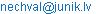(N. A. Nechval)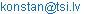(K. N. Nechval)

Nicholas A. Nechval, Konstantin N. Nechval. Tolerance Limits on Order Statistics in Future Samples Coming from the Two-Parameter Exponential Distribution. American Journal of Theoretical and Applied Statistics. Special Issue: Novel Ideas for Efficient Optimization of Statistical Decisions and Predictive Inferences under Parametric Uncertainty of Underlying Models with Applications. Vol. 5, No. 2-1, 2016, pp. 1-6. doi: 10.11648/j.ajtas.s.2016050201.11

Abstract: This paper presents an innovative approach to constructing lower and upper tolerance limits on order statistics in future samples. Attention is restricted to invariant families of distributions under parametric uncertainty. The approach used here emphasizes pivotal quantities relevant for obtaining tolerance factors and is applicable whenever the statistical problem is invariant under a group of transformations that acts transitively on the parameter space. It does not require the construction of any tables and is applicable whether the past data are complete or Type II censored. The proposed approach requires a quantile of the F distribution and is conceptually simple and easy to use. For illustration, the two-parameter exponential distribution is considered. A practical example is given.

Keywords: Order Statistics, F Distribution, Lower Tolerance Limit, Upper Tolerance Limit

1. Introduction

Statistical tolerance limits are another tool for making statistical inference on an unknown population. As opposed to a confidence limit that provides information concerning an unknown population parameter, a tolerance limit provides information on the entire population; to be specific, one-sided tolerance limit is expected to capture a certain proportion or more of the population, with a given confidence level. For example, an upper tolerance limit for a univariate population is such that with a given confidence level, a specified proportion or more of the population will fall below the limit. A lower tolerance limit satisfies similar conditions.

It is often desirable to have statistical tolerance limits available for the distributions used to describe time-to-failure data in reliability problems. For example, one might wish to know if at least a certain proportion, say b, of a manufactured product will operate at least T hours. This question can not usually be answered exactly, but it may be possible to determine a lower tolerance limit L(X1, …, Xn), based on a preliminary random sample (X1, …, Xn), such that one can say with a certain confidence g that at least 100b % of the product will operate longer than L(X1, …, Xn). Then reliability statements can be made based on L(X1, …, Xn), or, decisions can be reached by comparing L(X1, …, Xn) to T. Tolerance limits of the type mentioned above are considered in this paper. That is, if fq (x) denotes the density function of the parent population under consideration and if S is any statistic obtained from the preliminary random sample (X1, …, Xn) of that population, then L(S) is a lower g probability tolerance limit for proportion b if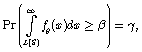(1)

and U(S) is an upper g probability tolerance limit for proportion b if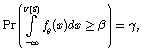(2)

where q is the parameter (in general, vector).

The common distributions used in life testing problems are the normal, exponential, Weibull, and gamma distributions . Tolerance limits for the normal distribution have been considered in , , , and others.

Tolerance limits enjoy a fairly rich history in the literature and have a very important role in engineering and manufacturing applications. Patel  provides a review (which was fairly comprehensive at the time of publication) of tolerance intervals for many distributions as well as a discussion of their relation with confidence intervals for percentiles and prediction intervals. Dunsmore  and Guenther, Patil, and Uppuluri  both discuss 2-parameter exponential tolerance intervals and the estimation procedure in greater detail. Engelhardt and Bain  discuss how to modify the formulas when dealing with type II censored data. Guenther  and Hahn and Meeker  discuss how one-sided tolerance limits can be used to obtain approximate two-sided tolerance intervals by applying Bonferroni's inequality.

In contrast to other statistical limits commonly used for statistical inference, the tolerance limits (especially for the order statistics) are used relatively rarely. One reason is that the theoretical concept and computational complexity of the tolerance limits is significantly more difficult than that of the standard confidence and prediction limits. Thus it becomes necessary to use the innovative approaches which will allow one to construct tolerance limits on future order statistics for many populations.

In this paper, the innovative approach to constructing lower and upper tolerance limits on order statistics in future samples is proposed. For illustration, the two-parameter exponential distribution is considered.

2. Mathematical Preliminaries

2.1. Probability Distribution Function of Order Statistic

Theorem 1. If there is a random sample of m ordered observations Y1££Ym from a known distribution (continuous or discrete) with density function fq (y), distribution function Fq (y), then the probability distribution function of the kth order statistic Yk, kÎ{1, 2, …, m}, is given by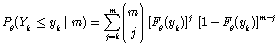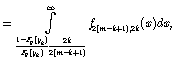(3)

where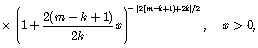(4)

is the probability density function of an F distribution with 2(m-k+1) and 2k degrees of freedom.

Proof. Suppose an event occurs with probability p per trial. It is well-known that the probability P of its occurring k or more times in m trials is termed a cumulative binomial probability, and is related to the incomplete beta function Ix(a, b) as follows: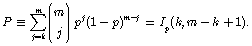(5)

It follows from (5) that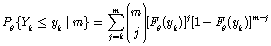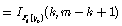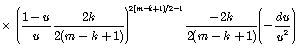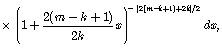(6)

where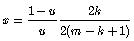(7)

This ends the proof.

Corollary 1.1.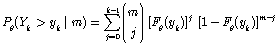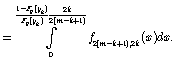(8)

Corollary 1.2. If yk,m;g is the quantile of order g for the distribution of Yk, we have from (3) that yk,m;g is the solution of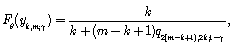(9)

where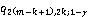is the quantile of order 1-g for the F distribution with 2(m-k+1) and 2k degrees of freedom.

2.2. Two-Parameter Exponential Distribution

The two-parameter exponential distribution is a widely used and widely known distribution. It is characterized by the density function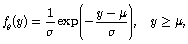(10)

where q = (m,s), s (scale parameter) and m (shift parameter) are unknown. The distribution function of the two-parameter exponential distribution is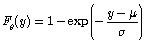(11)

The sufficient statistic for the parameter q, based on the r (£ n) smallest observations (X1 £. .. £ Xr) in a random sample of size n from the two-parameter exponential distribution (10) is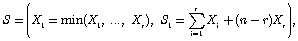(12)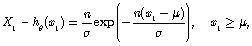(13)

where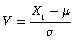(14)

is the pivotal quantity with the density function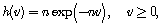(15)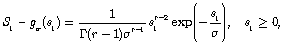(16)

where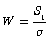(17)

is the pivotal quantity with the density function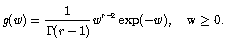(18)

3. Tolerance Limits for Order Statistic

3.1. Lover Tolerance Limit

Theorem 2. Let X1££Xr be the first r ordered observations from the preliminary sample of size n from a two-parameter exponential distribution defined by the density function (10). Then a lower one-sided b-content tolerance limit at level g, Lk ºLk (S) (on the kth order statistic Yk from a set of m future ordered observations Y1££Ym also from the distribution (10) ), which satisfies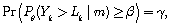(19)

is given by(20)

where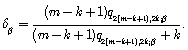(21)

Proof. It follows from (8), (11) and (19) that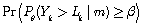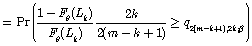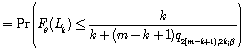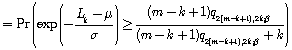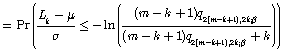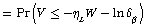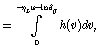(22)

where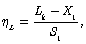(23)

is the lower tolerance factor,(24)

It follows from (18), (19) and (22) that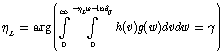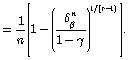(25)

Taking into account (23) and (25), we have (20). This completes the proof.

Corollary 2.1. If k=m=1, then(26)

The result similar to that of (26) can be found in [7,8].

3.2. Upper Tolerance Limit

Theorem 3. Let X1££Xr be the first r ordered observations from the preliminary sample of size n from a two-parameter exponential distribution defined by the density function (10). Then a lower one-sided b-content tolerance limit at level g, Uk ºUk (S) (on the kth order statistic Yk from a set of m future ordered observations Y1££Ym also from the distribution (10) ), which satisfies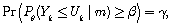(27)

is given by(28)

where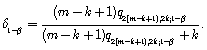(29)

Proof. It follows from (3), (11) and (27) that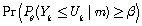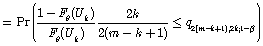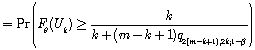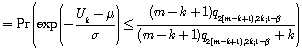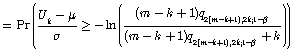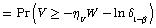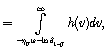(30)

where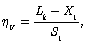(31)

is the upper tolerance factor,(32)

It follows from (18), (27) and (30) that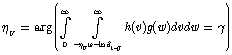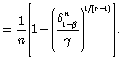(33)

Taking into account (31) and (33), we have (28). This completes the proof.

Corollary 3.1. If k=m=1, then(34)

The result similar to that of (34) can by found in .

Remark 1. It will be noted that an upper tolerance limit may be obtained from a lower tolerance limit by replacing b by 1-b, g by 1-g.

4. Practical Example

An industrial firm has the policy to replace a certain device, used at several locations in its plant, at the end of 24-month intervals. It doesn’t want too many of these items to fail before being replaced. Shipments of a lot of devices are made to each of three firms. Each firm selects a random sample of l= 5 items and accepts his shipment if no failures occur before a specified lifetime has accumulated. In order to find this specified lifetime, the manufacturer wishes to take a random sample of size n=15 and to calculate the lower one-sided simultaneous tolerance limit Lk=1(S) which is expected to capture a certain proportion b=0.95 or more of the population of selected items (m=3l), with a given confidence level g=0.95. This tolerance limit is such that one can say with a certain confidence g that at least 100b % of the product selected for testing by firms will operate longer than L1(S). The resulting lifetimes (rounded off to the nearest month) of the initial sample of size n = 15 from a population of the aforementioned devices are given in Table 1.

Table 1. The resulting lifetimes of the initial sample of size n=15.

 Observations (in terms of month intervals) X1 X2 X3 X4 X5 X6 X7 X8 8 9 10 12 14 17 20 25 X9 X10 X11 X12 X13 X14 X15 29 30 35 40 47 54 62

Goodness-of-fit testing. It is assumed that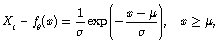(35)

where the parameters m and s are unknown. Thus, for this example, we have that r = n = 15, m = 3l =15, k = 1, b = 0.95, g = 0.95,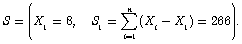(36)

It can be shown that the(37)

are i.i.d. U(0,1) rv’s (Nechval et al. ). We assess the statistical significance of departures from the two-parameter exponential model (10) by performing the Kolmogorov-Smirnov goodness-of-fit test. We use the K statistic (Muller et al. ). The rejection region for the a=0.05 level of significance is {K ³ Kn;a}. The percentage points for Kn;a were given by Muller et al. . For this example,

K = 0.280 < Kn=13;a=0.05 = 0.361.     (38)

Thus, there is not evidence to rule out the two-parameter exponential model.

Now the lower one-sided simultaneous b-content tolerance limit at the confidence level g, L1 ºL1 (S) (on the order statistic Y1 from a set of m future ordered observations Y1££Ym ) can be obtained from (20). Since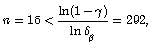(39)

where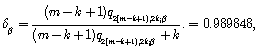(40)

it follows from (20) that(41)

Statistical inference. Thus, the manufacturer has 95% assurance that no failures will occur in the proportion b=0.95 or more of the population of selected items before L1 = 4 month intervals.

5. Conclusion

Tolerance limits enjoy a fairly rich history in the literature and have a very important role in engineering and manufacturing applications. In contrast to other statistical limits commonly used for statistical inference, the tolerance limits (especially for the order statistics) are used relatively rarely. One reason is that the theoretical concept and computational complexity of the tolerance limits is significantly more difficult than that of the standard confidence and prediction limits. Thus it becomes necessary to use the innovative approaches which will allow one to construct tolerance limits on future order statistics for many populations.

References

1. V. Mendenhall, "A bibliography on life testing and related topics," Biometrika, vol. XLV, pp. 521–543, 1958.
2. I. Guttman, "On the power of optimum tolerance regions when sampling from normal distributions," Annals of Mathematical Statistics, vol. XXVIII, pp. 773–778, 1957.
3. A. Wald and J. Wolfowitz, "Tolerance limits for a normal distribution," Annals of Mathematical Statistics, vol. XVII, pp. 208–215, 1946.
4. W. A. Wallis, "Tolerance intervals for linear regression," in Second Berkeley Symposium on Mathematical Statistics and Probability, University of California Press, Berkeley, 1951, pp. 43–51.
5. J. K. Patel, "Tolerance limits: a review," Communications in Statistics: Theory and Methodology, vol. 15, pp. 2719–2762, 1986.
6. I. R. Dunsmore, "Some approximations for tolerance factors for the two parameter exponential distribution," Technometrics, vol. 20, pp. 317–318, 1978.
7. W. C. Guenther, S. A. Patil, and V. R. R. Uppuluri, "One-sidedb-content tolerance factors for the two parameter exponential distribution," Technometrics, vol. 18, pp. 333–340, 1976.
8. M. Engelhardt and L.J. Bain, "Tolerance limits and confidence limits on reliability for the two-parameter exponential distribution," Technometrics, vol. 20, pp. 37–39, 1978.
9. W. C.Guenther, "Tolerance intervals for univariate distributions," Naval Research Logistics Quarterly, vol. 19, pp. 309–333, 1972.
10. G. J. Hahn and W. Q. Meeker, Statistical Intervals: A Guide for Practitioners. New York: John Wiley & Sons, 1991.
11. N.A. Nechval, and K.N. Nechval,"Characterization theorems for selecting the type of underlying distribution," in Proceedings of the 7th Vilnius Conference on Probability Theory and 22nd European Meeting of Statisticians. Vilnius: TEV, 1998, pp. 352– 353.
12. P. H. Muller, P. Neumann, and R. Storm,Tables of Mathematical Statistics. Leipzig: VEB Fachbuchverlag, 1979.

 Contents 1. 2. 2.1. 2.2. 3. 3.1. 3.2. 4. 5.
Article ToolsAbstractPDF(246K)